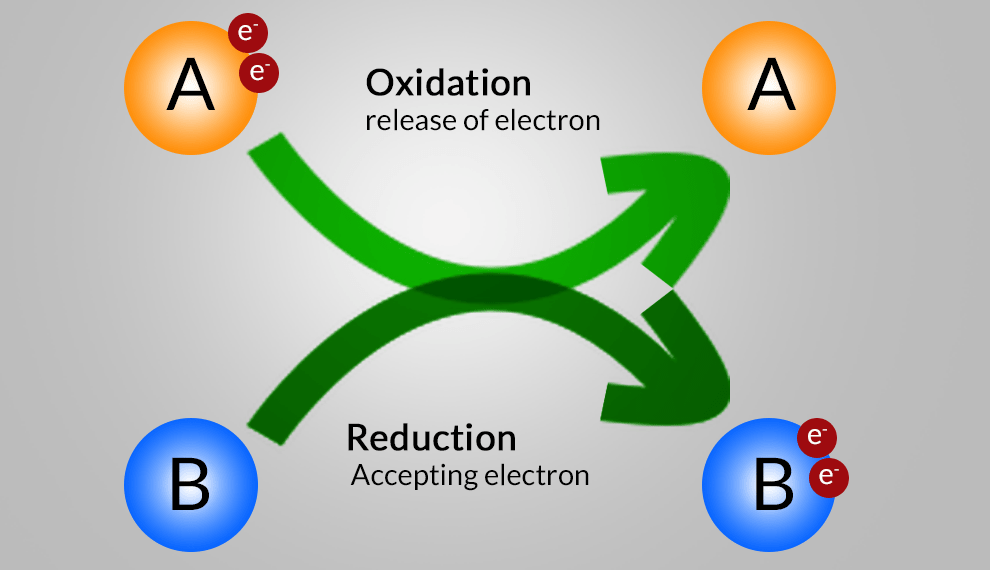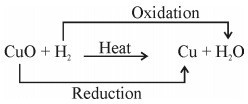# Chemical Reactions And Equations – Redox Reaction, Oxidation and Reduction ReactionsRedox Reaction, Oxidation and Reduction Reactions with exact equations

This part of the types of chemical reaction of chapter Chemical Reactions and Equations involve the types Redox Reaction, Oxidation and Reduction Reactions with exact equations.

v). Redox Reaction: A reaction in which reduction and oxidation takes place simultaneously.• ZnO (s)    +    C (s)      →   Zn (s)    +   CO (g)

Zinc              Carbon      Zinc           Carbon

Oxide                                                  Monoxide

vi). Oxidation Reaction: Oxidation is the gain of oxygen. For example:

• 2 Cu (s)   +    O2 (g)     →    2 CuO (s)  (Black)

Copper        Oxygen           Copper Oxide

•  2 Mg(s)    +   O2 (g)      →    2MgO (s)

Magnesium   Oxygen       Magnesium Oxide

OR

Removal of hydrogen is also oxidation. For Example:

•   2HI (g)          →    H2  (g)     +    I2 (g)

Hydrogen          Hydrogen    Iodine

Iodic Acid

VII). Reduction Reaction: Reduction is the gain of hydrogen. For example:

•   H2 (g)     +      Cl(g)  →    2 HCl

Hydrogen    Chlorine      Hydrochloric Acid

OR

Removal of oxygen is also reduction. Foe Example:

•   CuO (s)       +      CO (g)       →      Cu (s)     +     CO2 (g)

Copper Oxide   Carbon                Copper          Carbon Dioxide

(Black)             Monoxide           (Reddish Brown)

•   ZnO (s)    +    C (s)      →   Zn (s)    +   CO (g)

Zinc              Carbon        Zinc            Carbon

Oxide                                                     Monoxide

An oxidation-reduction (redox) reaction is a type of chemical reaction that involves a transfer of electrons between two species. An oxidation-reduction reaction is any chemical reaction in which the oxidation number of a molecule, atom, or ion changes by gaining or losing an electron. Redox Reaction, Oxidation and Reduction Reactions with exact equations are common and vital to some of the basic functions of life, including photosynthesis, respiration, combustion, and corrosion or rusting.

1.   Oxidation: The process in which there is loss of electrons.
2.   Reduction: The process in which there is gain of electrons.
3.   Oxidizing Agent: A substance that brings about oxidation.
4.  Reducing Agents: A substance that brings about reduction.

viii). Endothermic Reactions: Reaction in which heat is  absorbed to carry out the chemical reaction. For Example:

•        CaCO3  (s)      +      Heat      →      CaO (s)      +      CO2  (g)                                                                                                                           Calcium                                      Calcium           Carbon

Carbonate                                    Oxide               Dioxide

•   C(s)    +  H2 O (s)  +    Heat  →    CO (g)    +    H2 (g)

Carbon                                             Carbon

Monoxide (Evolve)

ix). Exothermic Reactions: Reaction in which heat is evolved during the chemical reaction. For Example:

•   C    +    O2    →     CO2 (g)    +     heat